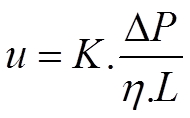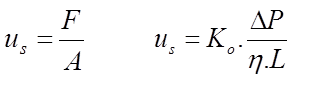## Permeability Equations - Definition

The definition of permeability of a packed (U)HPLC column is frequently inappropriately and incorrectly used in many popular and column manufacturer publications. This short article and the pages in the submenus are intended to clarify this area.

In prinicple, the permeability of a packed (U)HPLC column is defined as the proportionality factor in the relation between velocity of the solvent in the column and pressure drop.Here u is the average solvent velocity, ΔP the net pressure drop over the column, η the viscosity of the solvent and L the length of the column. The proportionality factor K, has the dimension m^2 .

Two questions however pop-up:

1. how is the solvent velocity defined?
2. how does K relate to the porous structure of the packed particle bed?

This will be addressed in the following pages of this blog.

## Permeability Equations - Superficial Velocity

The definitions provided here are derived from two manuscripts:

G. Deininger, Strömungvorgänge in Säulen und ihre Bedeutung in der Säulenchromatographie,
Ber. Bunsengesellschaft für physikalische Chemie, 77, 145 (1973).

C.A. Cramers, J.A. Rijks, J.P.M. Schutjes, Factors determining flow rate in chromatographic columns,
Chromatographia, 14, 439-444, (1981).

In chemical engineering the solvent velocity is defined as (lhs in equation 2.2). This is called the superficial velocity.F is the volumetric flow rate through the column and A the cross sectional surface of the empty column. In equation 2.2, Ko is called "specific permeability". This formulation is also known as Darcy's law.

However other definitions of solvent velocity are used in this context and need to be explained.